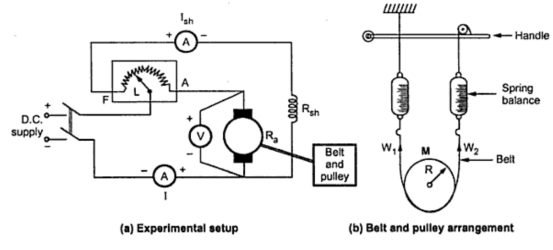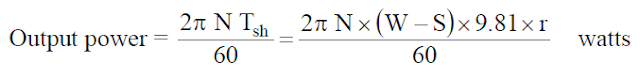0
1367

# Brake Test On DC Shunt Motor

## How to conduct brake test on dc shunt motors?

#### 5. The speed can be measured by a tachometer. Thus all the motor characteristics can be plotted.## Calculation Of  Brake Test On DC Shunt Motor

#### Hence the output power can be obtained as, Say speed of the pulley is N r.p.m., then, The above equation shows the output power of dc shunt motor in brake test.

Now let, V = Voltage applied in volts
I = Total line current drawn in amps.
As we know V,I am input parameters of dc motors in brake test.then,
Pin=V.I Watts  …..(3)
We have output and input.Then why late go and find the efficiency of dc shunt motor.
Efficiency (η)=Output/Input [No units]

#### From equation (2) & (3)## Disadvantages Of Brake Test On DC Shunt Motor

#### 5. The efficiency observed is on the lower side.

READ HERE  Measuring Electricity How energy monitors work